:   Netscrap(TM)   :   Trivia   :   Netscrap #737   :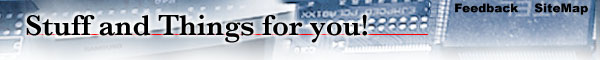Cool Test

```----- Begin NetScrap(TM) -----

Cool Test

This test does not measure intelligence, your fluency with words,
creativity or mathematical ability.  It will, however give you some
gauge of your mental flexibility. In the three years since the test
was developed, few people have been found who could solve more than
half of the 27 questions on the first try.

been set aside, particularly at unexpected moments when their minds
were relaxed. Some report solving all the questions over a period of
several days.

For example:
60 = M in an H
The answer would be: 60 = Minutes in an Hour

1. 26 = L of the A

2. 1,001 = A N

3. 7 = W of the W

4. 12 = S of the Z

5. 54 = C in a D (w/ the J)

6. 9 = P in the S S

7. 88 = K on a P

8. 13 = S on the A F

9. 32 = D F at which W F

10. 18 = H on a G C

11. 90 = D in a R A

12. 200 = D for P G in M

13. 8 = S on a S S

14. 3 = B M (S H T R)

15. 4 = Q in a G

16. 24 = H in a D

17. 1 = W on a U

18. 5 = D in a Z C

19. 57 = H V

20. 11 = P on a F T

21. 1,000 = W that a P is W

22. 29 = D in F in a L Y

23. 64 = S on a C

24. 15 = M on a D M C

25. 52 = W in a Y

26. 99 = B of B on the W

27. 3 = L P and one B B W

28. 30 = D H S

29. 5,280 = F in a M

30. 212 = D F at which W B

31. 10 = F in one L of B

32. 13 = N in a B d

33. 7 = D in S W (D, G, S, D, B, H & S)

34. 9 = L a C has

35. 40 = D and N it R (N and the A)

36. 25 = P in a Q

37. 3 = S (C. L, & M)

38. 50 = S in the U

39. 18 = W on a S T T

40. 3 = C in a F

41. 18 = W in a S

42. 10 = Y in a D

43. 15 = T D in A

44. 100 = Y in a C

45. 180 = D in a S L

46. 31 = D in J and J

47. 21 = A of C

48. 52 = W in a Y

49. 10 = D in a D

50. 50 = Q on T T

----- End NetScrap(TM) -----```
Entered on: 09/24/1998
 Send it: Claim it: Copy and paste this into an email to a friend. We can make it easy for you. Mail it off with the Netscrap(TM) MailTool. Did you do this? Do you own it? Can you prove it? Netscrap.com's mission is to reunite jokes like this with their creators. Take credit for your fine work.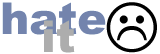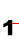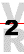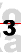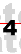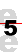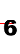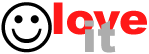```
---------------------------------------------------------------------------
75 Chars Wide```
We're testing ads. Send feedback if you have opinions about this.